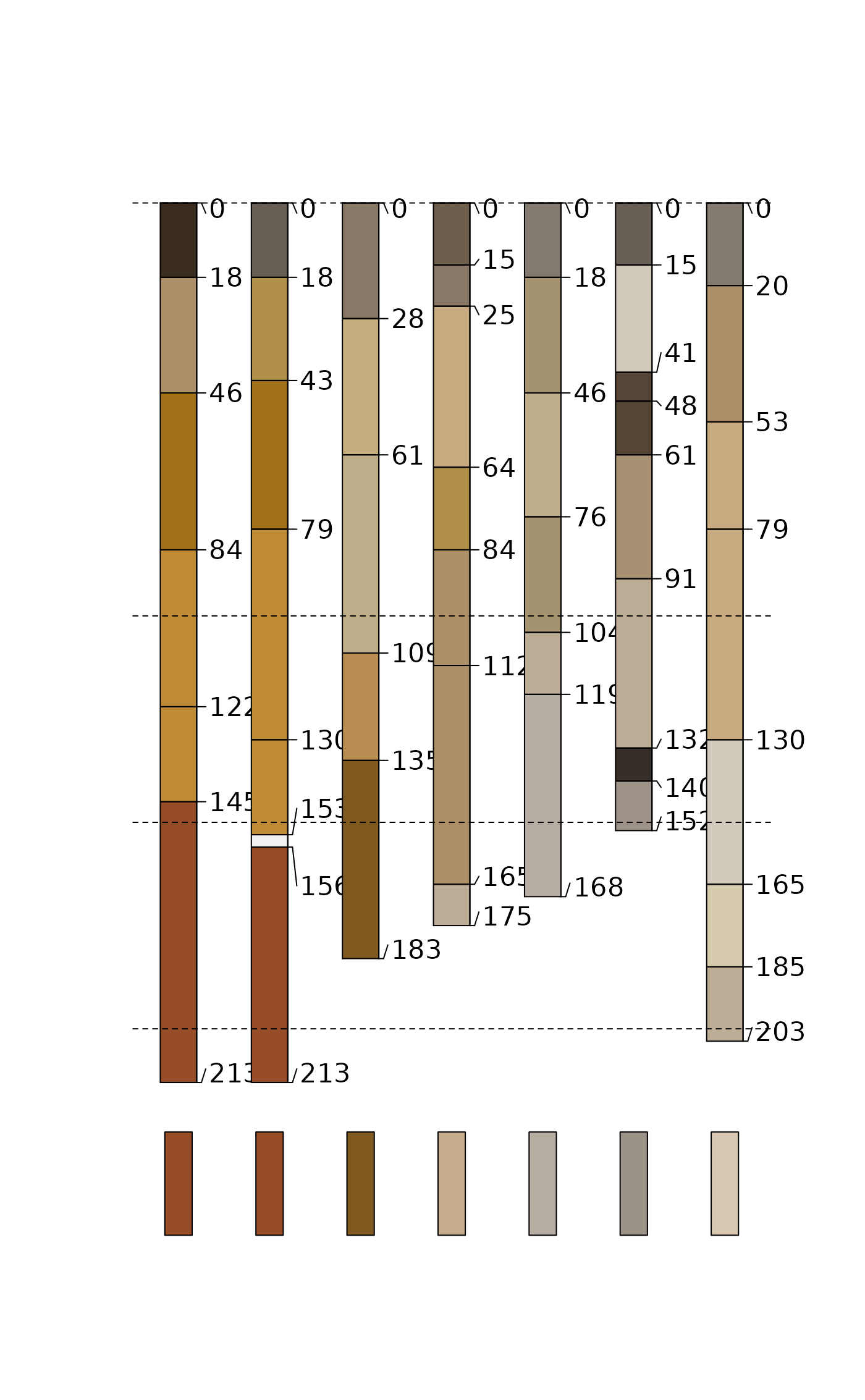Calculate "rubification" component of "Profile Development Index" after Harden (1982) "A quantitative index of soil development from field descriptions: Examples from a chronosequence in central California". Accepts vectorized inputs for hue and chroma to produce vector output.

In Harden (1982) "rubification" is calculated relative to a reference parent material. Several other non-color components are normalized relative to a maximum value and summed to obtain the overall Profile Development Index.

harden.rubification(hue, chroma, hue_ref, chroma_ref)

## Arguments

hue

A character vector containing Munsell hues (e.g. "7.5YR")

chroma

A numeric vector containing Munsell chromas

hue_ref

A character vector containing Munsell hue(s) (e.g. "10YR") for reference material

chroma_ref

A numeric vector containing Munsell chroma(s) for reference material

## Value

A numeric vector reflecting horizon redness increase relative to a reference (e.g. parent) material.

Andrew G. Brown

## Examples


# keep examples from using more than 2 cores

library(aqp)
data("jacobs2000", package="aqp")

# LEFT JOIN hue, value, chroma matrix color columns
horizons(jacobs2000) <- cbind(horizons(jacobs2000)[,c(idname(jacobs2000), hzidname(jacobs2000))],
parseMunsell(jacobs2000$matrix_color_munsell, convertColors = FALSE)) #' # calculate a mixed 150-200cm color ~"parent material" jacobs2000$c_horizon_color <- profileApply(jacobs2000, function(p) {

# and derive the parent material from the 150-200cm interval
p150_200 <- glom(p, 150, 200, truncate = TRUE)
p150_200$thickness <- p150_200$bottom - p150_200$top # subset colors and thickness clrs <- na.omit(horizons(p150_200)[,c('matrix_color_munsell','thickness')]) # simulate a subtractive mixture using thickness as weight mixMunsell( clrs$matrix_color_munsell,
w = clrs$thickness, mixingMethod = 'exact')$munsell
})

# segment profile into 1cm slices (for proper depth weighting)
jacobs2000$rubif <- profileApply(jacobs2000, function(p) { # sum the melanization index over the 0-100cm interval p0_100 <- segment(p, 0:100) ccol <- parseMunsell(p$c_horizon_color, convertColors = FALSE)

sum(harden.rubification(
hue = p0_100$hue, chroma = as.numeric(p0_100$chroma),
hue_ref = ccol$hue, chroma_ref = as.numeric(ccol$chroma)
), na.rm = TRUE)

})

jacobs2000$rubiforder <- order(jacobs2000$rubif)

# Plot in order of increasing Rubification index

plotSPC(jacobs2000,
color = "matrix_color",
label = "rubif",
plot.order = jacobs2000$rubiforder, max.depth = 250 ) segments( x0 = 0.5, x1 = length(jacobs2000) + 0.5, y0 = c(0,100,150,200), y1 = c(0,100,150,200), lty = 2 ) # Add [estimated] parent material color swatches trash <- sapply(seq_along(jacobs2000$c_horizon_color), function(i) {
rect(i - 0.15, 250, i + 0.15, 225,
col = parseMunsell(jacobs2000$c_horizon_color[jacobs2000$rubiforder[i]]))
})